# Lions Weekly: January 4, 2023# A Note From Mr. Sandefur

Lion Family,

Welcome back! I pray that each of you had a wonderful Christmas and a time of break and relaxation. As you are well aware, the break was a bit of an active one on our campus as our team worked diligently to prepare for the upcoming semester. I want to take the opportunity to thank our Facilities and Food Services teams publicly for all of their hard work in adjusting to the challenges that occurred on campus with the frozen water lines. Here at TKA, we are a family, and that sentiment rang true during this season of difficulty and opportunity.

I also would like to take the time to encourage your family to prayerfully consider the priority re-enrollment period that opened yesterday. This current academic year has been an incredible one in terms of student achievement, enrollment numbers, etc., and planning for next year has already begun in earnest. In order to plan accordingly our priority re-enrollment window is crucial in the life of The Academy. Please consider saving your student's spot during this month in order to save on the re-enrollment fee and to allow school staff the ability to plan accordingly. We are truly grateful for your partnership and are prayerfully looking forward to many years of developing servant leaders that will change the world for Christ.

Jeremy Sandefur

 table div table+table+table+table div table{width:100%;padding:0}table div table+table+table+table div table img{width:96.23%;padding:0;float:none}table div table+table+table+table div table td{width:100%;padding:0 1.88% 18px}/* styles */table div table+table+table+table+table+table div table{width:100%;padding:0}table div table+table+table+table+table+table div table img{width:96.23%;padding:0;float:none}table div table+table+table+table+table+table div table td{width:100%;padding:0 1.88% 18px}/* styles */# Priority Re-enrollment is Now Open

We hope that 2023 is off to a wonderful start as we look forward to all that God has in store for The King’s Academy in the coming months. Thank you for the privilege of partnering with you to equip your child to impact the world for Christ!

Re-enrollment for the 2023-2024 school year is now open! To assist our administrative team in planning for the coming year, it is vital that all current students re-enroll as soon as possible. As an incentive, we are offering an early enrollment discount to everyone who submits re-enrollment through February 3. The regular re-enrollment fee of \$300 per student will be reduced to only \$100 per student if re-enrollment is completed by February 3, 2023!
Click the following link to complete your re-enrollment form and make payment:

Please note that re-enrollment CANNOT be completed on a smartphone or tablet.

## Instructions for completing re-enrollment:

Please note that this form looks different than previous years as we are working to streamline this process as much as possible.

• After logging in, click on the “Apply/Enroll” tab on the left, then click “Enrollment/Reenrollment.”

• Follow the instructions on the screen to complete the re-enrollment packet. Please be sure to update any information that has changed over the past year such as address, insurance, and phone numbers.

• Once all information is updated and complete, FACTS will ask for the re-enrollment fee.

Please remember that re-enrollment is for current TKA students only. Any siblings of current students or new students who have not been enrolled at our school prior to this point will need to start an application found on our website.

Contact the Admissions and Enrollment Office by phone or email if you have any questions or need help with the re-enrollment process. We hope to see all of our families return to TKA next year! We are thankful for the opportunity to partner with you in the education and development of your students.

Sincerely,

Lori Campbell
(865) 573-8321 option 1

## Pertinent information regarding the 2023-24 academic year can be found by utilizing the below listed links: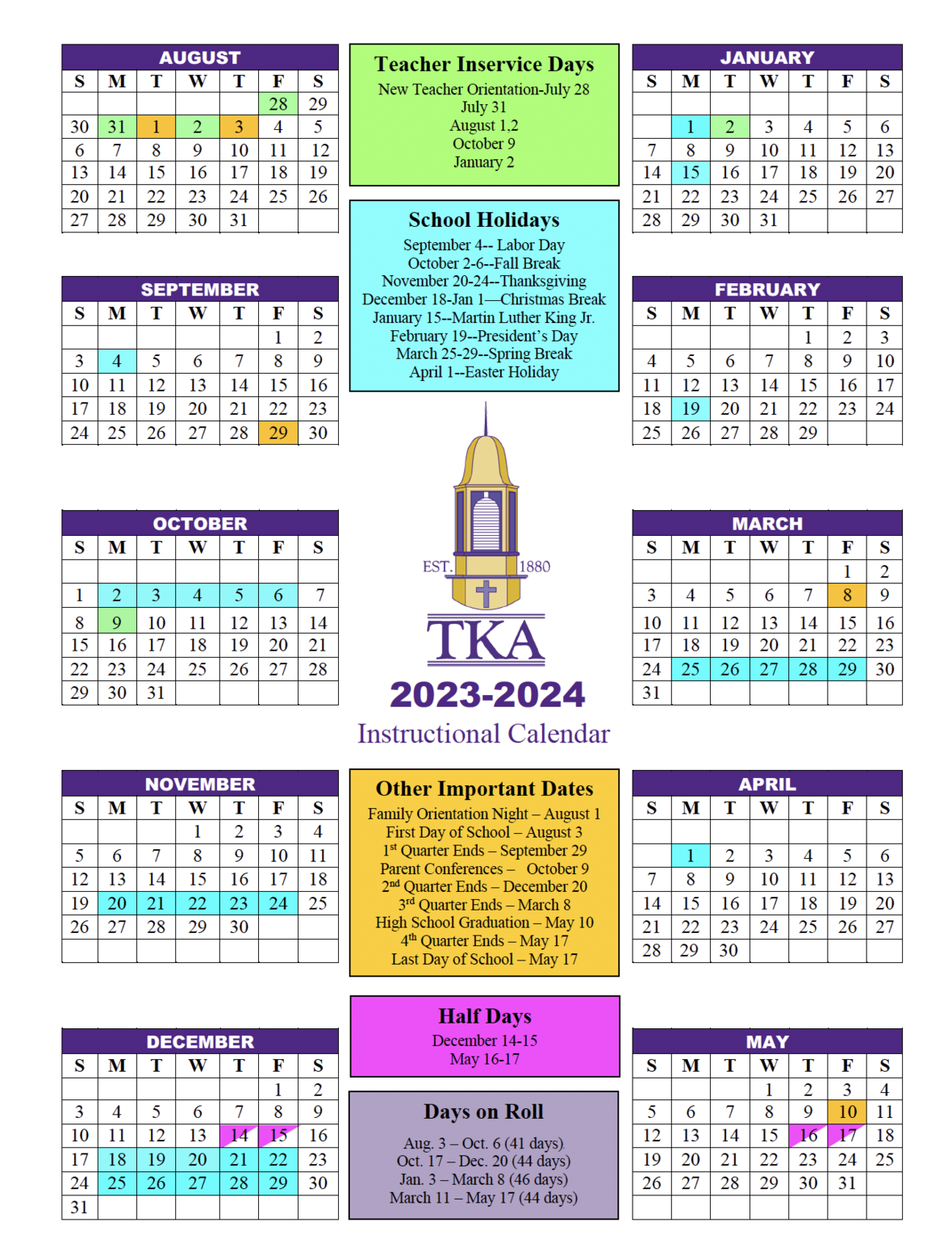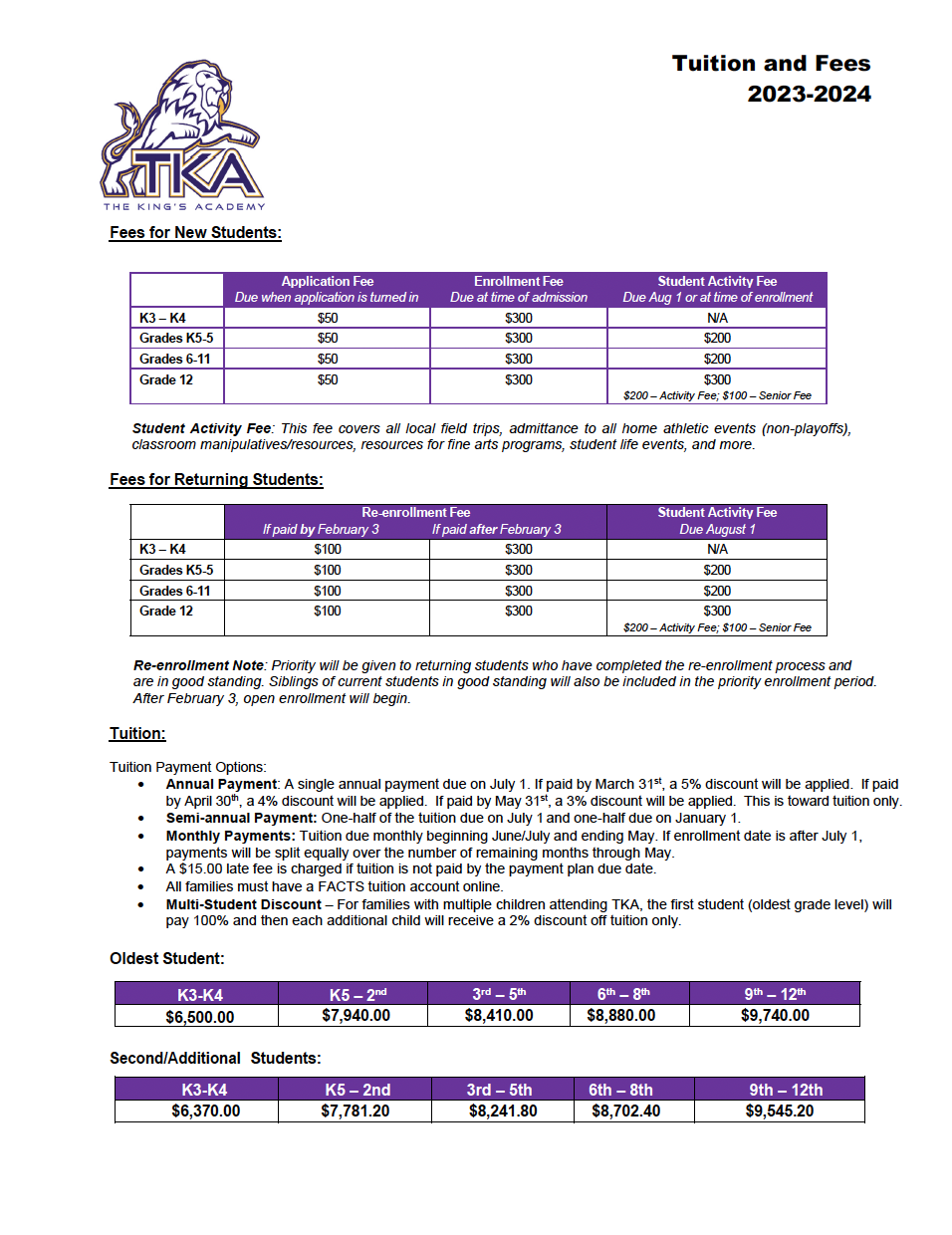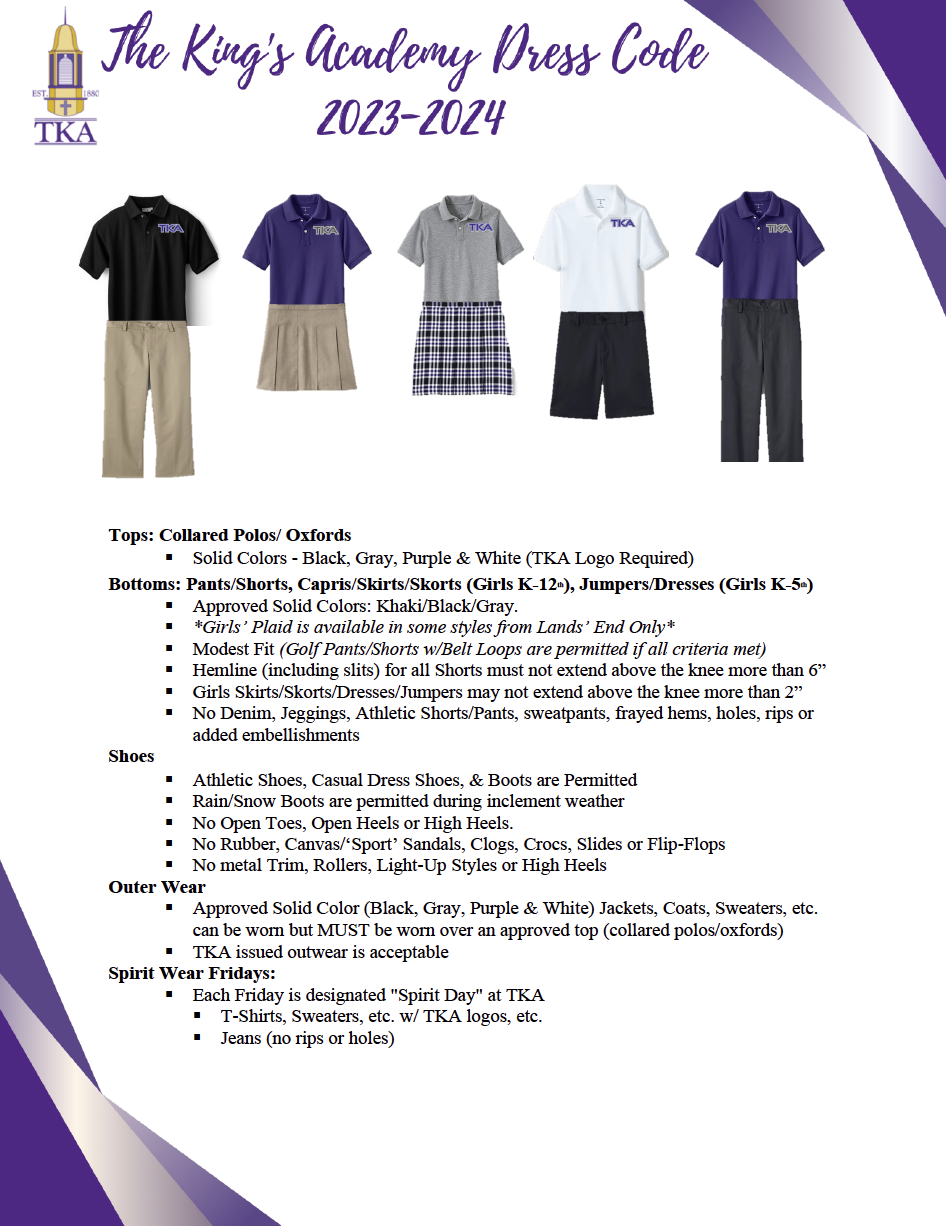table div table+table+table+table+table+table+table+table+table+table+table+table+table+table+table+table+table div table{width:100%;padding:0}table div table+table+table+table+table+table+table+table+table+table+table+table+table+table+table+table+table div table img{width:96.23%;padding:0;float:none}table div table+table+table+table+table+table+table+table+table+table+table+table+table+table+table+table+table div table td{width:100%;padding:0 1.88% 18px}/* styles */# Campus Information Update

Below is some information regarding the changes that you will notice next week:

Ashe Cafeteria
Due to the damage that occurred in the actual kitchen (the seating area of the cafeteria was completely unimpacted), we will be cooking our school meals at Seymour First Baptist Church and transporting them to be served in the cafeteria at students' regularly scheduled lunch times. We are grateful to SFBC as well as our Culinary Services team for their flexibility in ensuring that our students are provided meals while the kitchen is repaired. While we only expect this arrangement to be needed for a week or two, your child will see slight modifications in menu selections due to the need to prepare meals offsite. Our Culinary Services team is working diligently to ensure that all student needs are taken care of during this brief season of disruption.

Schubert Dormitory
Due to the damage that has occurred inside Schubert Dormitory, our Residence Life and Leadership Team is working diligently to move students to Stewart Dormitory until the necessary work is completed (carpet, ceiling tiles replaced, etc.). We are truly grateful for the volunteers who assisted this week in washing all student sheets, rugs, etc. that were affected in preparing for their return. Students are expected to be in this new rooming configuration for a few short weeks and will return to their previous space once all work is completed.

With Great Appreciation
I want to thank Derek McNutt, Johnny Cupp, Christopher Moore, Tyler Rhoton, and Kendedrick Jones, along with all others who have helped, for working tirelessly since Christmas Eve when issues began on campus. These dedicated servants have spent hours of their vacation time to ensure that our students start school in as normal of an environment as possible. When you see one of these amazing members of our TKA family, please thank them for their hard work and dedication.

While these incidents are not ideal, we are grateful for the Lord's protection and provision. Our Lion family has answered the call of service quickly and with love, for which I am truly grateful. I am anxiously awaiting the start of the Spring Semester on January 3rd, and am greatly looking forward to seeing your students back on campus very soon. We greatly appreciate your prayers, support, and understanding as we continue to address these occurrences that are outside of our control.

 table div table+table+table+table+table+table+table+table+table+table+table+table+table+table+table+table+table+table+table div table{width:100%;padding:0}table div table+table+table+table+table+table+table+table+table+table+table+table+table+table+table+table+table+table+table div table img{width:96.23%;padding:0;float:none}table div table+table+table+table+table+table+table+table+table+table+table+table+table+table+table+table+table+table+table div table td{width:100%;padding:0 1.88% 18px}/* styles */# High School Tennis

High School Tennis season is just around the corner! Any high school students interested in playing, please make plans to attend the meeting on Wednesday, January 11, at 3:10 p.m. in Brandes.

Contact Coach Jenny Cook 865-387-3468 with any questions.

 table div table+table+table+table+table+table+table+table+table+table+table+table+table+table+table+table+table+table+table+table+table div table{width:100%;padding:0}table div table+table+table+table+table+table+table+table+table+table+table+table+table+table+table+table+table+table+table+table+table div table img{width:96.23%;padding:0;float:none}table div table+table+table+table+table+table+table+table+table+table+table+table+table+table+table+table+table+table+table+table+table div table td{width:100%;padding:0 1.88% 18px}/* styles */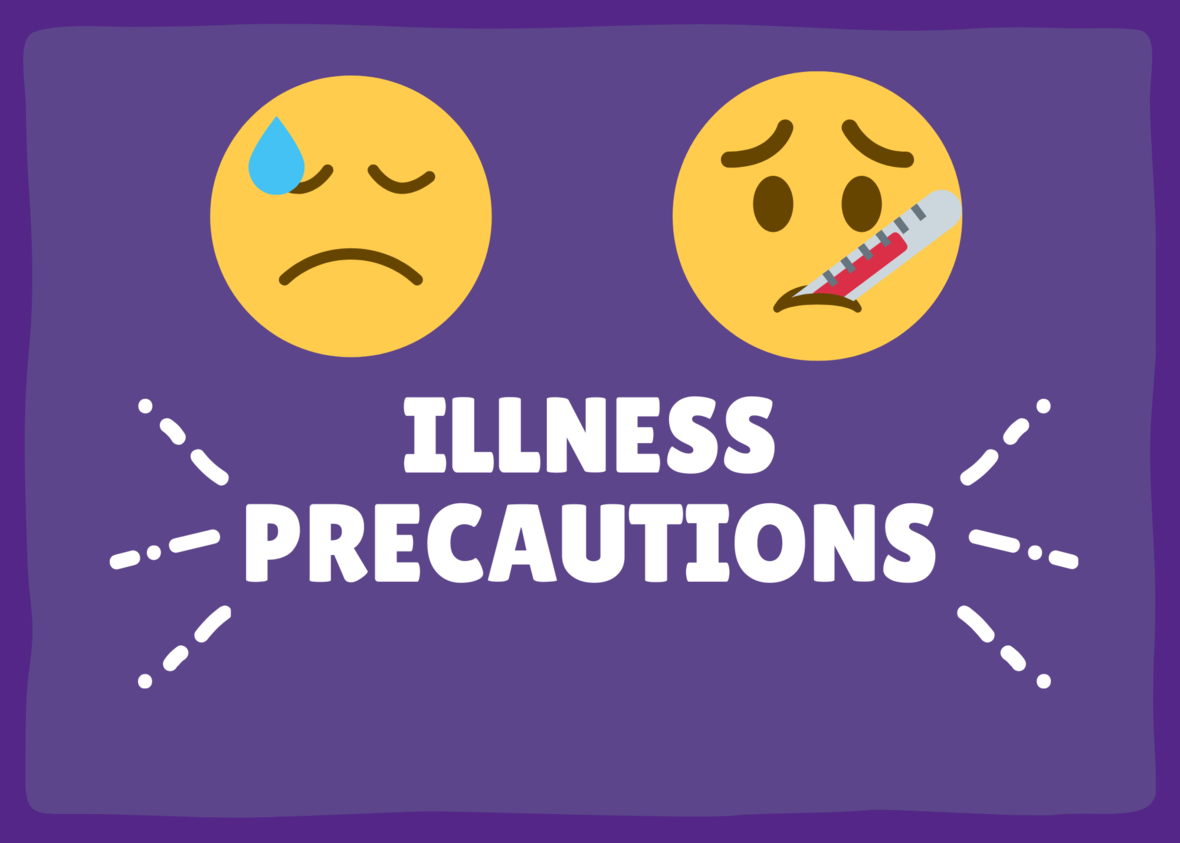# Illness Precautions

As we enter into cold and flu season, we need your help to mitigate the potential spread of illness by monitoring symptoms and keeping your child home if they present with a fever or are sick with other symptoms. Teachers and administrators will work with students to make up work in a timely manner once they are feeling better. By partnering with us, we will strive together to stay as healthy as possible during this season.

 table div table+table+table+table+table+table+table+table+table+table+table+table+table+table+table+table+table+table+table+table+table+table+table div table{width:100%;padding:0}table div table+table+table+table+table+table+table+table+table+table+table+table+table+table+table+table+table+table+table+table+table+table+table div table img{width:96.23%;padding:0;float:none}table div table+table+table+table+table+table+table+table+table+table+table+table+table+table+table+table+table+table+table+table+table+table+table div table td{width:100%;padding:0 1.88% 18px}/* styles */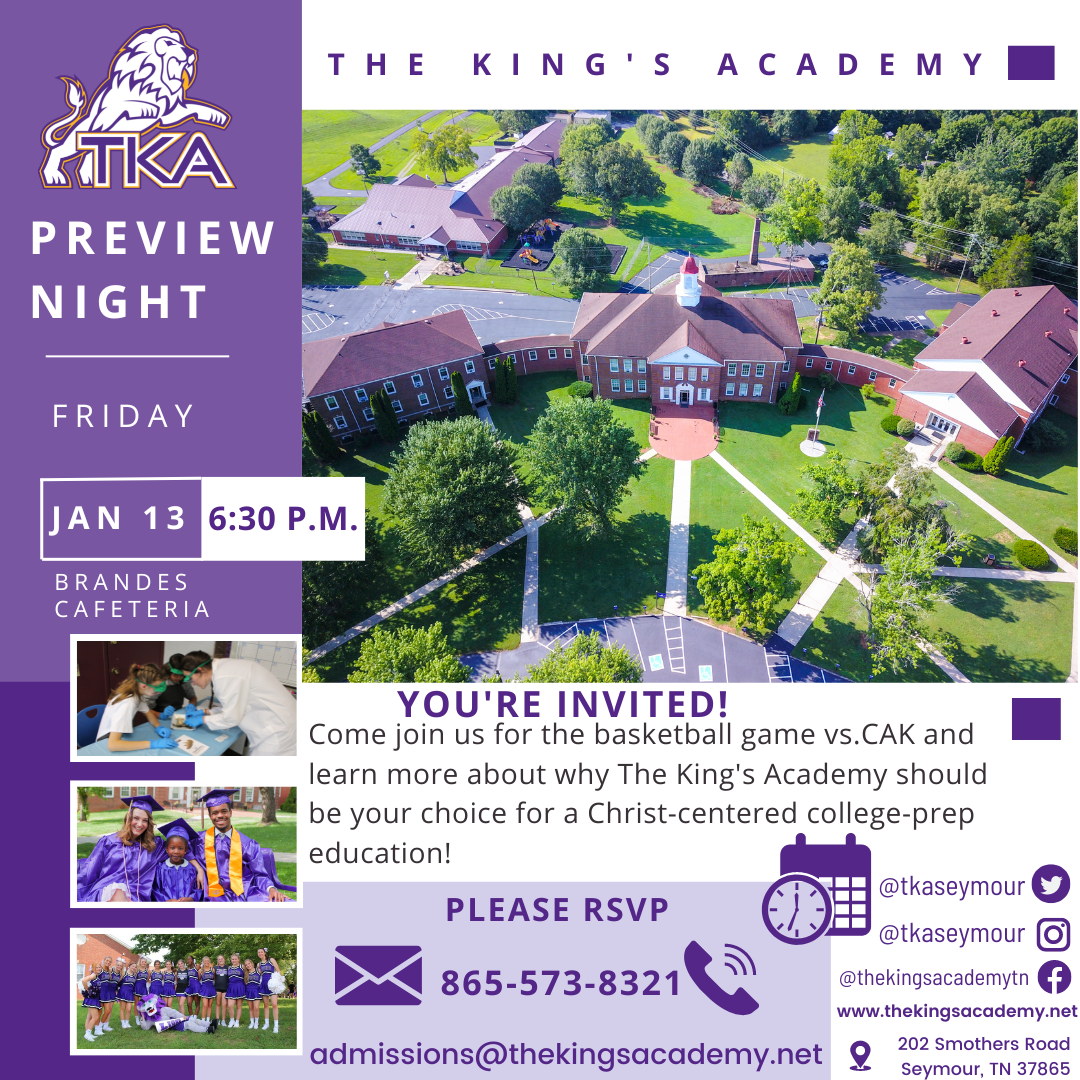# Preview Night

## Friday, January 13

Do you have a friend or family member who would be interested in learning more about TKA?

TKA admissions will host a prospective student preview night on Friday, January 13, at 6:30 p.m. in Brandes.

Friends or family interested in possibly sending a new student to TKA may RSVP by clicking the link below, emailing admissions@thekingsacademy.net, or calling at 865-573-8321.

 table div table+table+table+table+table+table+table+table+table+table+table+table+table+table+table+table+table+table+table+table+table+table+table+table+table+table div table{width:100%;padding:0}table div table+table+table+table+table+table+table+table+table+table+table+table+table+table+table+table+table+table+table+table+table+table+table+table+table+table div table img{width:96.23%;padding:0;float:none}table div table+table+table+table+table+table+table+table+table+table+table+table+table+table+table+table+table+table+table+table+table+table+table+table+table+table div table td{width:100%;padding:0 1.88% 18px}/* styles */table div table+table+table+table+table+table+table+table+table+table+table+table+table+table+table+table+table+table+table+table+table+table+table+table+table+table+table+table div table{width:100%;padding:0}table div table+table+table+table+table+table+table+table+table+table+table+table+table+table+table+table+table+table+table+table+table+table+table+table+table+table+table+table div table img{width:96.23%;padding:0;float:none}table div table+table+table+table+table+table+table+table+table+table+table+table+table+table+table+table+table+table+table+table+table+table+table+table+table+table+table+table div table td{width:100%;padding:0 1.88% 18px}/* styles */table div table+table+table+table+table+table+table+table+table+table+table+table+table+table+table+table+table+table+table+table+table+table+table+table+table+table+table+table+table+table div table{width:100%;padding:0}table div table+table+table+table+table+table+table+table+table+table+table+table+table+table+table+table+table+table+table+table+table+table+table+table+table+table+table+table+table+table div table img{width:96.23%;padding:0;float:none}table div table+table+table+table+table+table+table+table+table+table+table+table+table+table+table+table+table+table+table+table+table+table+table+table+table+table+table+table+table+table div table td{width:100%;padding:0 1.88% 18px}/* styles */# We're Hiring!

TKA has an employment opportunity available in food services.

Food Services Employee: TKA is seeking an employee to faithfully serve in our food services department. Prior food service experience is preferred. Please email resumes to HR@thekingsacademy.net or call 865-573-8321.

 table div table+table+table+table+table+table+table+table+table+table+table+table+table+table+table+table+table+table+table+table+table+table+table+table+table+table+table+table+table+table+table+table+table div table{width:100%;padding:0}table div table+table+table+table+table+table+table+table+table+table+table+table+table+table+table+table+table+table+table+table+table+table+table+table+table+table+table+table+table+table+table+table+table div table img{width:96.23%;padding:0;float:none}table div table+table+table+table+table+table+table+table+table+table+table+table+table+table+table+table+table+table+table+table+table+table+table+table+table+table+table+table+table+table+table+table+table div table td{width:100%;padding:0 1.88% 18px}/* styles */# D1 Training

I hope the holiday season has been wonderful for you all. I am happy to announce that we will be adding morning workout times in the near future. The times added will be a 6 am and 7 am class for adults. We understand time is important, and we want to make sure that we help and assist you as much as we can in order for you to reach your health goals. Many times resolutions fail because of a lack of accountability- That's why D1 is here! To keep you accountable to yourself, your family, and your commitment. Join the team!

Melvin Jones
D1 Sevierville
865-719-9421table div table+table+table+table+table+table+table+table+table+table+table+table+table+table+table+table+table+table+table+table+table+table+table+table+table+table+table+table+table+table+table+table+table+table+table+table div table{width:100%;padding:0}table div table+table+table+table+table+table+table+table+table+table+table+table+table+table+table+table+table+table+table+table+table+table+table+table+table+table+table+table+table+table+table+table+table+table+table+table div table img{width:96.23%;padding:0;float:none}table div table+table+table+table+table+table+table+table+table+table+table+table+table+table+table+table+table+table+table+table+table+table+table+table+table+table+table+table+table+table+table+table+table+table+table+table div table td{width:100%;padding:0 1.88% 18px}/* styles */# 2022-23 Yearbooks

2022-23 yearbooks are now on sale! Scan this QR code to visit the site to purchase yours today. For any questions regarding yearbooks, contact Mr. Josh Smith at jsmith@thekingsacademy.net.

 table div table+table+table+table+table+table+table+table+table+table+table+table+table+table+table+table+table+table+table+table+table+table+table+table+table+table+table+table+table+table+table+table+table+table+table+table+table+table div table{width:100%;padding:0}table div table+table+table+table+table+table+table+table+table+table+table+table+table+table+table+table+table+table+table+table+table+table+table+table+table+table+table+table+table+table+table+table+table+table+table+table+table+table div table img{width:96.23%;padding:0;float:none}table div table+table+table+table+table+table+table+table+table+table+table+table+table+table+table+table+table+table+table+table+table+table+table+table+table+table+table+table+table+table+table+table+table+table+table+table+table+table div table td{width:100%;padding:0 1.88% 18px}/* styles */# 9-12th Spring Break Trip: France, Germany and The Alps

## March 11-18, 2023

 table div table+table+table+table+table+table+table+table+table+table+table+table+table+table+table+table+table+table+table+table+table+table+table+table+table+table+table+table+table+table+table+table+table+table+table+table+table+table+table+table div table{width:100%;padding:0}table div table+table+table+table+table+table+table+table+table+table+table+table+table+table+table+table+table+table+table+table+table+table+table+table+table+table+table+table+table+table+table+table+table+table+table+table+table+table+table+table div table img{width:96.23%;padding:0;float:none}table div table+table+table+table+table+table+table+table+table+table+table+table+table+table+table+table+table+table+table+table+table+table+table+table+table+table+table+table+table+table+table+table+table+table+table+table+table+table+table+table div table td{width:100%;padding:0 1.88% 18px}/* styles */# Weekly Sports Scheduletable.module-42{width:0%;padding:0}table div table+table+table+table+table+table+table+table+table+table+table+table+table+table+table+table+table+table+table+table+table+table+table+table+table+table+table+table+table+table+table+table+table+table+table+table+table+table+table+table+table+table+table div table{width:0%;float:none;margin-left:auto;margin-right:auto;padding:0}table div table+table+table+table+table+table+table+table+table+table+table+table+table+table+table+table+table+table+table+table+table+table+table+table+table+table+table+table+table+table+table+table+table+table+table+table+table+table+table+table+table+table+table div table a{border:0 none;text-decoration:none}table div table+table+table+table+table+table+table+table+table+table+table+table+table+table+table+table+table+table+table+table+table+table+table+table+table+table+table+table+table+table+table+table+table+table+table+table+table+table+table+table+table+table+table div table img{width:100%!important;border:0 none;text-decoration:none}table div table+table+table+table+table+table+table+table+table+table+table+table+table+table+table+table+table+table+table+table+table+table+table+table+table+table+table+table+table+table+table+table+table+table+table+table+table+table+table+table+table+table+table div table td{width:100%;padding:0}/* styles */
 table div table+table+table+table+table+table+table+table+table+table+table+table+table+table+table+table+table+table+table+table+table+table+table+table+table+table+table+table+table+table+table+table+table+table+table+table+table+table+table+table+table+table+table+table div table{width:100%;padding:0}table div table+table+table+table+table+table+table+table+table+table+table+table+table+table+table+table+table+table+table+table+table+table+table+table+table+table+table+table+table+table+table+table+table+table+table+table+table+table+table+table+table+table+table+table div table img{width:96.23%;padding:0;float:none}table div table+table+table+table+table+table+table+table+table+table+table+table+table+table+table+table+table+table+table+table+table+table+table+table+table+table+table+table+table+table+table+table+table+table+table+table+table+table+table+table+table+table+table+table div table td{width:100%;padding:0 1.88% 18px}/* styles */# Important information for the 2022-2023 School Year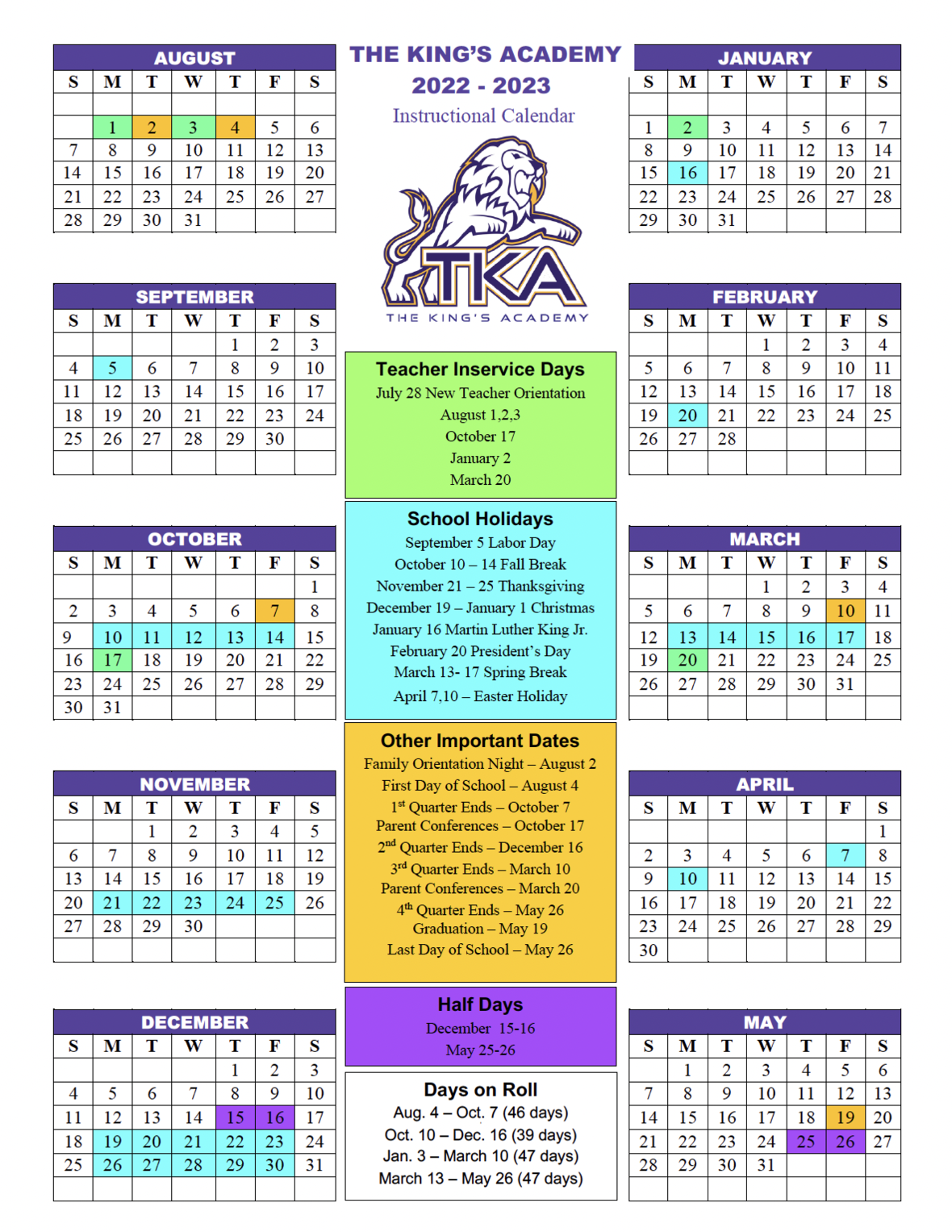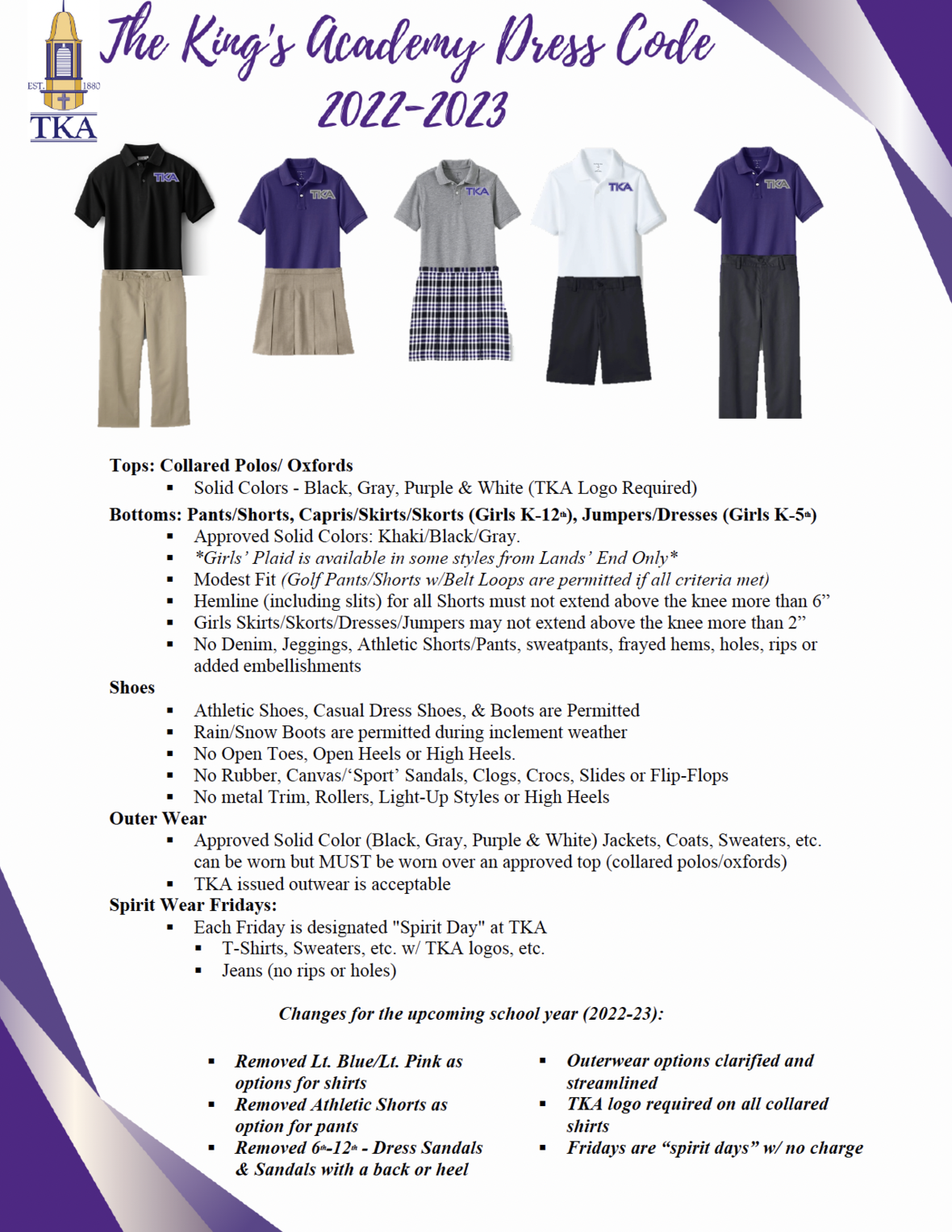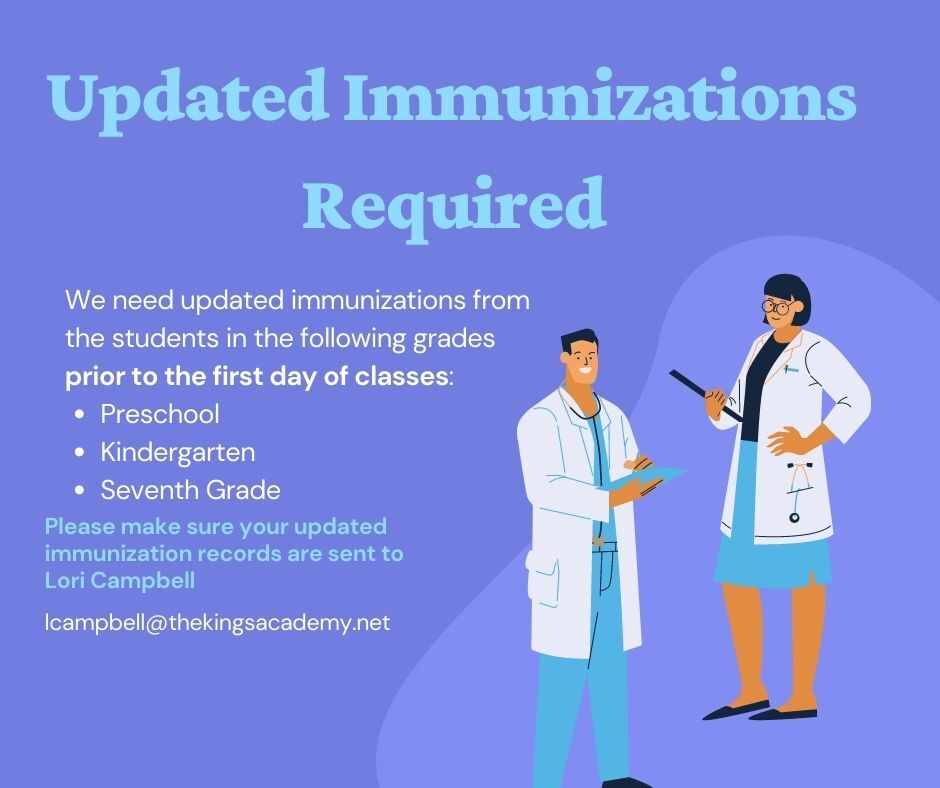table div table+table+table+table+table+table+table+table+table+table+table+table+table+table+table+table+table+table+table+table+table+table+table+table+table+table+table+table+table+table+table+table+table+table+table+table+table+table+table+table+table+table+table+table+table+table+table+table+table div table{width:100%;padding:0}table div table+table+table+table+table+table+table+table+table+table+table+table+table+table+table+table+table+table+table+table+table+table+table+table+table+table+table+table+table+table+table+table+table+table+table+table+table+table+table+table+table+table+table+table+table+table+table+table+table div table img{width:96.23%;padding:0;float:none}table div table+table+table+table+table+table+table+table+table+table+table+table+table+table+table+table+table+table+table+table+table+table+table+table+table+table+table+table+table+table+table+table+table+table+table+table+table+table+table+table+table+table+table+table+table+table+table+table+table div table td{width:100%;padding:0 1.88% 18px}/* styles */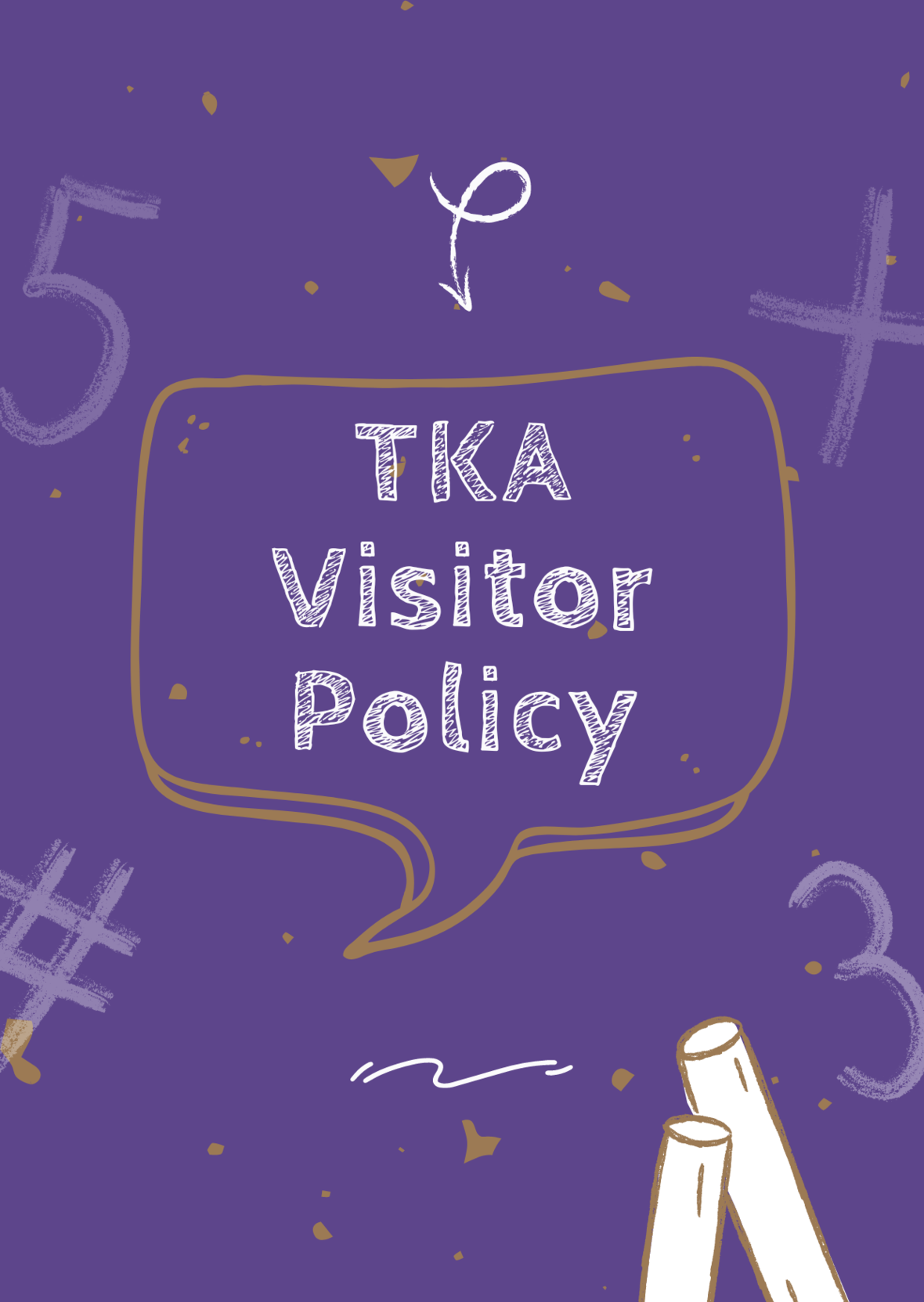# TKA Visitor Policy

In order to help maintain a safe and instructionally based learning environment for our students, all visitors for middle and high school must enter through the front door of the Anderson Building and sign in in the middle/high school office in order to receive a visitor's pass. All visitors for elementary must enter the front door of the Ogle Building in order to receive a visitor's pass. This pass should be visible at all times so that TKA faculty and staff know that you have checked in at the office.

Our doors are open to visitors, but please call ahead of time to schedule conferences with your child’s teachers. This will prevent interruptions to instruction and the loss of the valuable learning time of our students. Adults will not be allowed to wander the halls of the building or hold conferences with teachers during instructional times.

TKA family lunch visitors should follow the above procedure. Lunch tickets are \$5 each.

Students who are not enrolled at TKA are not permitted to visit TKA students during the school day, which includes lunch.

This policy has been developed in order to help maintain a safe and instruction focused environment at school each day. Any visitor who does not meet these expectations may be asked to leave school grounds.

 table div table+table+table+table+table+table+table+table+table+table+table+table+table+table+table+table+table+table+table+table+table+table+table+table+table+table+table+table+table+table+table+table+table+table+table+table+table+table+table+table+table+table+table+table+table+table+table+table+table+table+table div table{width:100%;padding:0}table div table+table+table+table+table+table+table+table+table+table+table+table+table+table+table+table+table+table+table+table+table+table+table+table+table+table+table+table+table+table+table+table+table+table+table+table+table+table+table+table+table+table+table+table+table+table+table+table+table+table+table div table img{width:96.23%;padding:0;float:none}table div table+table+table+table+table+table+table+table+table+table+table+table+table+table+table+table+table+table+table+table+table+table+table+table+table+table+table+table+table+table+table+table+table+table+table+table+table+table+table+table+table+table+table+table+table+table+table+table+table+table+table div table td{width:100%;padding:0 1.88% 18px}/* styles *//* styles */
 table div table+table+table+table+table+table+table+table+table+table+table+table+table+table+table+table+table+table+table+table+table+table+table+table+table+table+table+table+table+table+table+table+table+table+table+table+table+table+table+table+table+table+table+table+table+table+table+table+table+table+table+table+table+table div table{width:100%;padding:0}table div table+table+table+table+table+table+table+table+table+table+table+table+table+table+table+table+table+table+table+table+table+table+table+table+table+table+table+table+table+table+table+table+table+table+table+table+table+table+table+table+table+table+table+table+table+table+table+table+table+table+table+table+table+table div table img{width:96.23%;padding:0;float:none}table div table+table+table+table+table+table+table+table+table+table+table+table+table+table+table+table+table+table+table+table+table+table+table+table+table+table+table+table+table+table+table+table+table+table+table+table+table+table+table+table+table+table+table+table+table+table+table+table+table+table+table+table+table+table div table td{width:100%;padding:0 1.88% 18px}/* styles */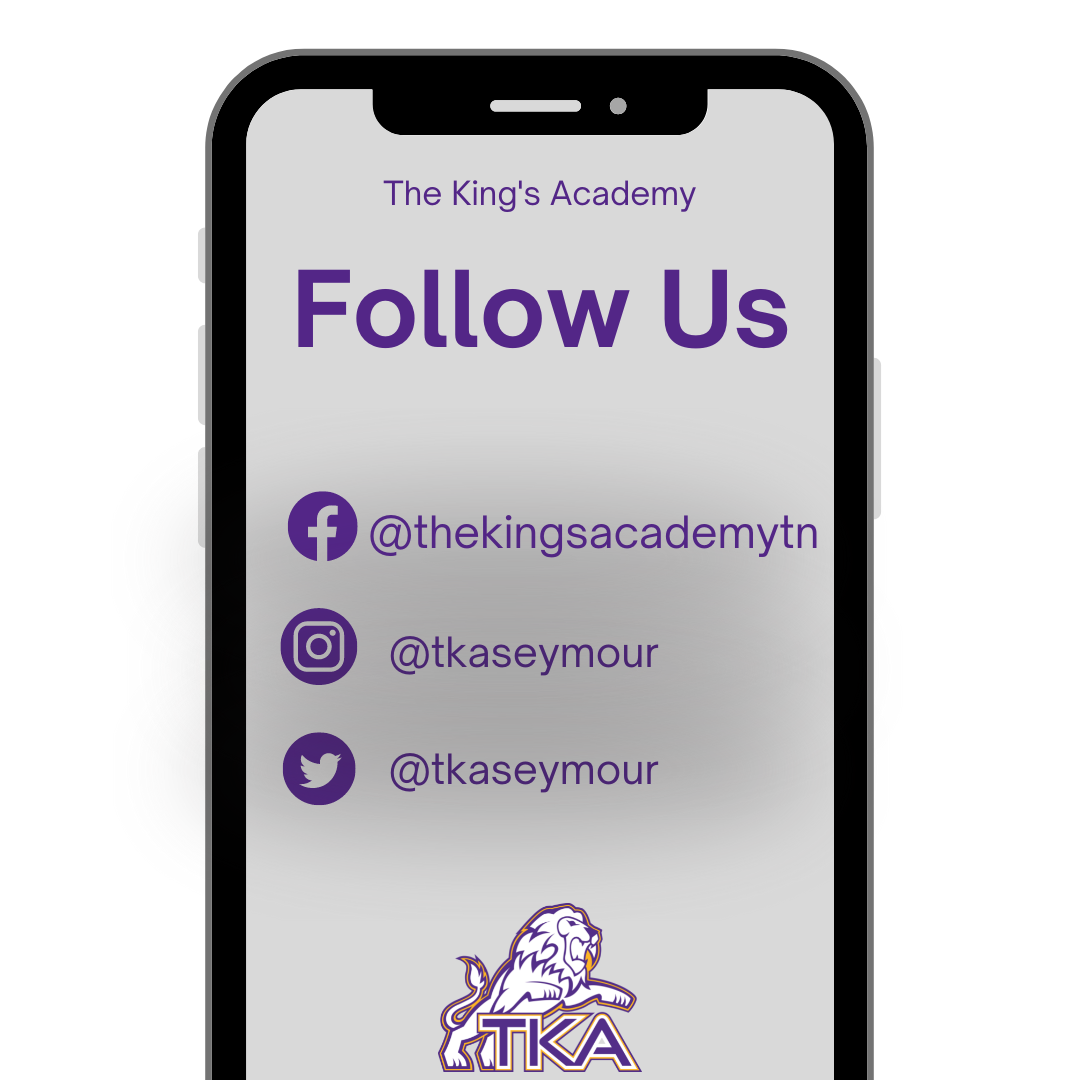table div table+table+table+table+table+table+table+table+table+table+table+table+table+table+table+table+table+table+table+table+table+table+table+table+table+table+table+table+table+table+table+table+table+table+table+table+table+table+table+table+table+table+table+table+table+table+table+table+table+table+table+table+table+table+table+table div table{width:100%;padding:0}table div table+table+table+table+table+table+table+table+table+table+table+table+table+table+table+table+table+table+table+table+table+table+table+table+table+table+table+table+table+table+table+table+table+table+table+table+table+table+table+table+table+table+table+table+table+table+table+table+table+table+table+table+table+table+table+table div table img{width:96.23%;padding:0;float:none}table div table+table+table+table+table+table+table+table+table+table+table+table+table+table+table+table+table+table+table+table+table+table+table+table+table+table+table+table+table+table+table+table+table+table+table+table+table+table+table+table+table+table+table+table+table+table+table+table+table+table+table+table+table+table+table+table div table td{width:100%;padding:0 1.88% 18px}/* styles *//* styles */ The King's Academy
 Like   Tweet